Mathematical Expression of Yarn Irregularity
Home Fabric Engineering Unevenness and Coefficient of Variation of Yarn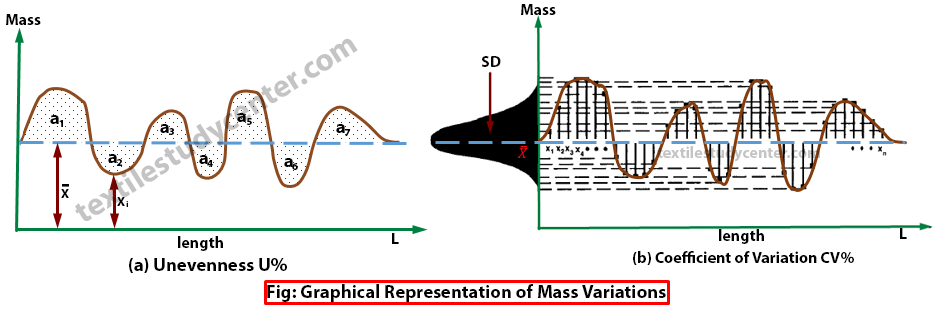# Unevenness and Coefficient of Variation of Yarn

8.23K
0#### Md Sohanur Rahman Sobuj

Co-founder , Admin & Author at Textile Study Center
Student of Bangladesh University of Textiles (BUTEX) Department: Apparel Engineering## Unevenness and Coefficient of Variation of Yarn

Yarn Evenness-1   Yarn Evenness-2   Yarn Evenness-3  Yarn Evenness-4

Mathematical Expression of Yarn Irregularity or Mass Variation

Generally two parameters are used to express the irregularity of yarns. They are…

• 1. Percentage of mean deviation (PMD) or Unevenness (U%)
• 2. Coefficient of variation CV%

Percentage of Mean Deviation (PMD) or Unevenness (U%)

The average value for all the deviations from the mean which is expressed as a percentage of the overall mean is called percentage of Mean Deviation (PMD). This is termed Um% by the Uster Company.

Coefficient of mass Variation (CV%):

The coefficient of mass variation CV % is the ratio of standard deviation of mass variation divided by average mass variation.The higher the CV value is the more irregular the yarn.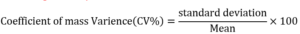A modern instrument, such as the Uster Evenness Tester, can measure the U and CV values of a fiber assembly at a high speed. The larger deviations from the mean value are much more intensively taken into consideration in the calculation of CV% rather than in U% (due to the squaring of the term). For this reason, the Coefficient of Variation CV% has received more recognition in
modern statistics than the irregularity value U%.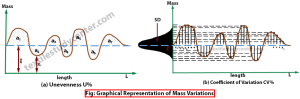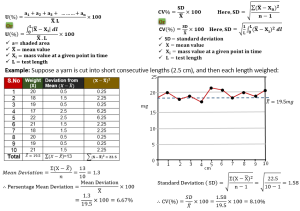Relation between Percentage of Mean Deviation (PMD) and Coefficient of Variation CV%

If the fiber assembly required to be tested in normally distributed with respect to the mass variation, then the two parameters of irregularity are related by the following equation: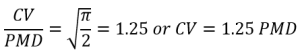Type of Fiber Assembly Conversion Factor ►Normal Distribution (contains purely random variations, symmetrical) ►Asymmetrical Distribution, periodic and random   variations present (faulty test material e.g. with long wavelength count variations, thick places, etc.) ►Symmetric Distribution with strong periodic variations 1.25   >1.25   <1.25 viagraforhealthyman

(8226)#### Md Sohanur Rahman Sobuj

Co-founder , Admin & Author at Textile Study Center
Student of Bangladesh University of Textiles (BUTEX) Department: Apparel Engineering#### Latest posts by Md Sohanur Rahman Sobuj (see all)Close
Share via